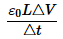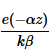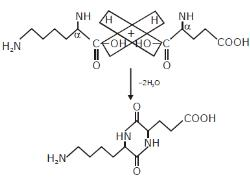Courses

# Previous Year Questions (JEE Advanced)

## 10 Questions MCQ Test | Previous Year Questions (JEE Advanced)

Description
This mock test of Previous Year Questions (JEE Advanced) for helps you for every entrance exam. This contains 10 Multiple Choice Questions for Previous Year Questions (JEE Advanced) (mcq) to study with solutions a complete question bank. The solved questions answers in this Previous Year Questions (JEE Advanced) quiz give you a good mix of easy questions and tough questions. students definitely take this Previous Year Questions (JEE Advanced) exercise for a better result in the exam. You can find other Previous Year Questions (JEE Advanced) extra questions, long questions & short questions for on EduRev as well by searching above.
*Multiple options can be correct
QUESTION: 1

Solution:
QUESTION: 2

### In the formula X = 3YZ2, X and Z have dimensions of capacitance and magnetic induction respectively. What are the dimensions of Y in MKSQ system ?                [JEE-1995,2/100]

Solution:
*Multiple options can be correct
QUESTION: 3

### Which of the following pairs have same dimensions :                     [JEE-1996' 2/100]

Solution:
*Multiple options can be correct
QUESTION: 4

The SI unit of inductance, the henry can be written as  :            [JEE-1998' 2/200]

Solution:
*Multiple options can be correct
QUESTION: 5

Let [ε0] denote the dimensional formula of the permittivity of the vaccum, and [μ0] that of the permeability of the vacuum. If M = mass, L = length, T = time and I = electric current  :               [JEE-1998' 2/200]

Solution:
QUESTION: 6

The dimensions of 1/2 ε0E2 (ε0 : permittivity of free space, E : electric field ) is :     [JEE Sc 2000' 2/200]

Solution:
QUESTION: 7

A quantity X is given by. where ε0 is the permittivity of free space, L is length, ΔV is potential difference and Δt is time interval. The dimensional formula for X is the same as that of    [JEE Sc.2000'3/105]

Solution:
QUESTION: 8

Pressure depends on distance as, P = α/β exp, where α,β are constants, z is distance,  k is Boltzmann's constant and θ is temperature. The dimension of β are      [JEE-2004s '3/84]

Solution:
QUESTION: 9

Which of the following set have different dimensions ?

Solution:
QUESTION: 10

A dense collection of equal number of electrons and positive ions is called neutral plasma. Certain solids containing fixed positive ions surrounded by free electrons can be treated as neutral plasma. Let 'N' be the number density of free electrons, each of mass 'm'. When the electrons are subjected to an electric field, they are displaced relatively away from the heavy positive ions. If the electric field becomes zero, the electrons begin to oscillate about the positive ions with a natural angular frequency 'ωp' which is called the plasma frequency. To sustain the oscillations, a time varying electric field needs to be applied that has an angular frequency ω, where a part of the energy is absorbed and a part of it is reflected. As ω approaches ωp, all the free electrons are set to resonance together and all the energy is reflected. This is the explanation of high reflectivity of metals.Taking the electronic charge as 'e' and the permittivity as 'ε0 ', use dimensional analysis to determine the correct expression for ωp.

[JEE-2011]

Solution:

Track your progress, build streaks, highlight & save important lessons and more!

### Similar Content### Related tests